##### Chemistry Workbook For Dummies with Online Practice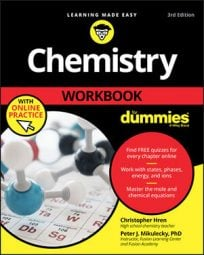A solid understanding of molality helps you to calculate changes in boiling and freezing points. In the same way, a solid understanding of boiling point elevation and freezing point depression can help you determine the molecular mass of a mystery compound that's being added to a known quantity of solvent.

Boiling point elevation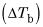refers to the tendency of a solvent's boiling point to increase when an impurity (a solute) is added to it.

Freezing point depression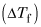refers to the tendency of a solvent's freezing point to decrease when an impurity is added.

## Calculate the molecular mass

When you're asked to solve problems of this type, you'll always be given the mass of the mystery solute, the mass of solvent, and either the change in the freezing or boiling point or the new freezing or boiling point itself. From this information, you then follow a set of simple steps to determine the molecular mass:
1. Find the boiling point elevation or freezing point depression.

If you've been given the boiling point, calculate theby subtracting the boiling point of the pure solvent from the number you were given. If you know the freezing point, subtract the freezing point of the pure solvent to it to get the2. Look up the Kb or Kf of the solvent (refer to the tables following this list).

3. Solve for the molality of the solution using the equation for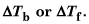4. Calculate the number of moles of solute in the solution by multiplying the molality calculated in Step 3 by the given number of kilograms of solvent.

5. Divide the given mass of solute by the number of moles calculated in Step 4.

This is your molecular mass, or number of grams per mole, from which you can often guess the identity of the mystery compound.

Common Kb Values
Solvent Kb in Degrees C/m Boiling Point in Degrees C
Acetic acid 3.07 118.1
Benzene 2.53 80.1
Camphor 5.95 204.0
Carbon tetrachloride 4.95 76.7
Cyclohexane 2.79 80.7
Ethanol 1.19 78.4
Phenol 3.56 181.7
Water 0.512 100.0
Common Kf Values
Solvent Kf in Degrees C/m Freezing Point in Degrees C
Acetic acid 3.90 16.6
Benzene 5.12 5.5
Camphor 37.7 179.0
Carbon tetrachloride 30.0 –23.0
Cyclohexane 20.2 6.4
Ethanol 1.99 –114.6
Phenol 7.40 41.0
Water 1.86 0.0
Here's an example: 97.30 g of a mystery compound is added to 500.0 g of water, raising its boiling point to 100.78 degrees C. What is the molecular mass of the mystery compound?

First subtract the boiling point of water from this new boiling point: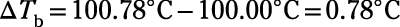Then plug this value and a Kb of 0.512 into the equation for boiling point elevation and solve for molality: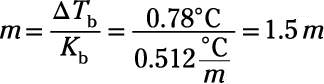Next, take this molality value and multiply it by the given mass of the solvent, water, in kilograms: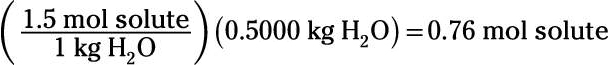Last, divide the number of grams of the mystery solute by the number of moles, giving you the molecular mass of the compound: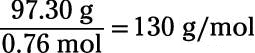The molecular mass of the mystery compound is 130 g/mol.